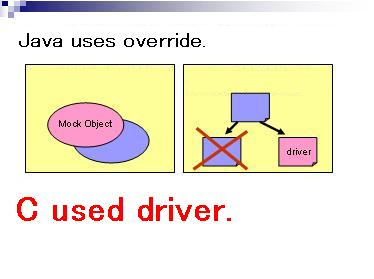# How to.

JUnit (Java testing framework) is used for "override" for unit testing, but "CUnit for Mr.Ando" is "stub" for unit testing.Fig.1 -- CUnit for Mr.Ando testing image.

## Test Sample.

But the easy usage is as follows. It is checking twice that a and b are equivalent in testEasySample().
``````#include <stdio.h>
#include <testRunner.h> /* ... (1) */

static unsigned int testEasySample(void);
static unsigned int testManyFunction(void);

/** Main function. */
int main(void) {
return (int) testRunner(testManyFunction); /* ... (2) */
}

unsigned int testEasySample(void) { /* ... (3) */
int a = 1 + 1;
int b = 2;

TEST_ASSERT_EQUALS(a,b); /* .... (4) */

return 0; /* ... (5) */
}

unsigned int testManyFunction(void) {

TEST_ASSERT(! testEasySample()); /* ... (6) */
TEST_ASSERT(! testEasySample());

return 0;
}``````
1. A test file needs to include testRunner.h and stdio.h. A program needs to compile and link testRunner.c.
2. In order to test, testRunner() is described in a main() sentence. The argument of testRunner() describes a test function.
3. The argument of a test function is a void type. A test function returns unsigned int.
"0" is a success. "Except 0" is failure.
4. As for this sample, 1+1 checks that it is 2.
TEST_ASSERT_EQUALS(,) is used when an equal result is desired.
TEST_ASSERT_NOT_EQUALS(,) is used when a not equal result is desired.
When an answer is mistaken, the contents are displayed and the return of the function is carried out.
5. Finally a function returns 0.
6. When you want to test by separating into two functions, it indicates. When the contents of TEST_ASSERT() are 0,test was failure. In this example,testEasySample() is carried out twice.
7. The result of a test is only displayed by O.K. or NG.
This is because anyone can check whether the test has been performed correctly.
Write once,run anyway and run anywhere.
When this library is employed, MUST NOT include these test codes in the object file to test. A test code and the code for a test should divide.
The separated sample is shown below. They are three different fundamental patterns, sequence, selection, and a foreach.
If such techniques are mastered, anyone can do the test of higher quality.